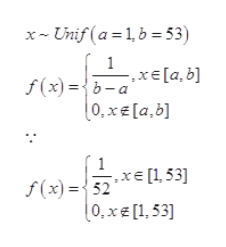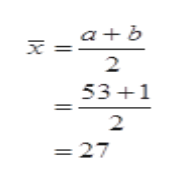Births are approximately uniformly distributed between the 52 weeks of the year. They can be said to follow a Uniform Distribution from 1 – 53 (spread of 52 weeks). Round all answers to two decimal places.A. The mean of this distribution isB. The standard deviation isC. The probability that a person will be born at the exact moment that week 29 begins is P(x = 29) =D. The probability that a person will be born between weeks 5 and 18 is P(5 < x < 18) =E. The probability that a person will be born after week 30 is P(x > 30) =F. P(x > 17 | x < 21) =G. Find the 40th percentile.H. Find the minimum for the upper quartile.

Question

Births are approximately uniformly distributed between the 52 weeks of the year. They can be said to follow a Uniform Distribution from 1 – 53 (spread of 52 weeks). Round all answers to two decimal places.

A. The mean of this distribution is

B. The standard deviation is

C. The probability that a person will be born at the exact moment that week 29 begins is P(x = 29) =

D. The probability that a person will be born between weeks 5 and 18 is P(5 < x < 18) =

E. The probability that a person will be born after week 30 is P(x > 30) =

F. P(x > 17 | x < 21) =

G. Find the 40th percentile.

H. Find the minimum for the upper quartile.

Step 1

Let x be random variable that follows uniform distribution between the 52 weeks that is,

Step 2help_outlineImage TranscriptionclosexUnif (a 1,b 53) f(x)={b-a 0, xe[a,b] 1 f(x)52x (0, x€ [1,53]| fullscreen
Step 3

a)

The mean of this distrib...help_outlineImage Transcriptionclosea b 2 53 1 2 =27 fullscreen

Want to see the full answer?

See Solution

Want to see this answer and more?

Our solutions are written by experts, many with advanced degrees, and available 24/7

See Solution
Tagged in

Statistics